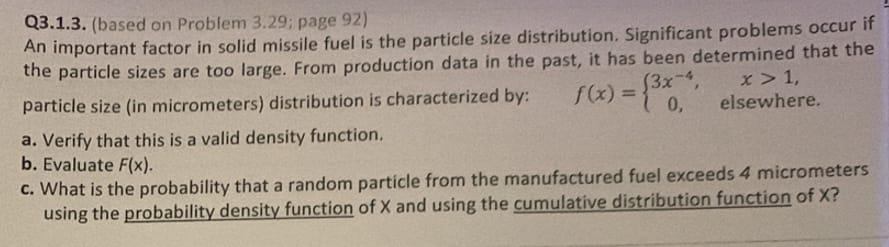Home / Expert Answers / Statistics and Probability / q3-1-3-based-on-problem-3-29-page-92-an-important-factor-in-solid-missile-fuel-is-the-particle-pa498

# (Solved): Q3.1.3. (based on Problem 3.29; page 92) An important factor in solid missile fuel is the particle ...Q3.1.3. (based on Problem 3.29; page 92) An important factor in solid missile fuel is the particle size distribution. Significant problems occur if the particle sizes are too large. From production data in the past, it has been determined that the particle size (in micrometers) distribution is characterized by: $$\quad f(x)=\left\{\begin{array}{cc}3 x^{-4}, & x>1, \\ 0, & \text { elsewhere. }\end{array}\right.$$ a. Verify that this is a valid density function. b. Evaluate $$F(x)$$. c. What is the probability that a random particle from the manufactured fuel exceeds 4 micrometers using the probability density function of $$X$$ and using the cumulative distribution function of $$X$$ ?

We have an Answer from Expert